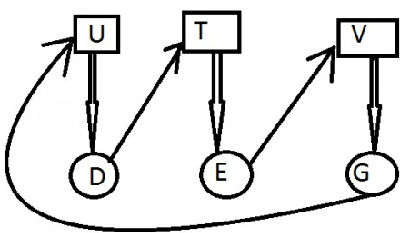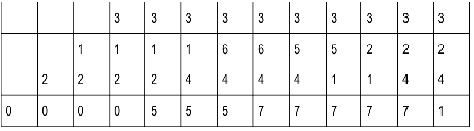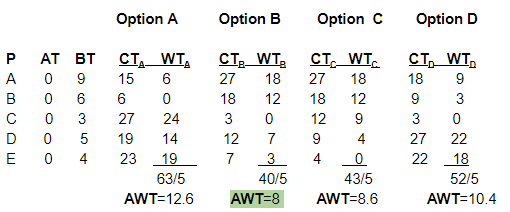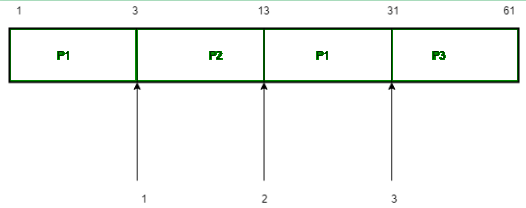# UGC NET COMPUTER SCIENCE SOLVED PAPERS 2014-16 - UGC NET Computer Science Paper 2 August 2016

>>>>>>>>UGC NET Computer Science Paper 2 August 2016

• A

No• B

Yes, A, B, C• C

Yes, D, E, G• D

Yes, A, B, F• Option : C
• Explanation :
System is in Deadlock due to process D,E,G
So option C• A

13• B

12• C

11• D

10• Option : A
• Explanation :So, option (A) is correct.

• A

70• B

14• C

13• D

7• Option : B
• Explanation : To avoid deadlock 'm' >= peak demands(P1 + P2 + P3) i.e. m >= peak demands(2 + 5 + 7) m >= peak demands(14) So, option (B) is correct.

• A

B, A, D, E, C• B

C, E, D, B, A• C

E, D, C, B, A• D

C, B, A, E, D• Option : B
• Explanation :
We will solve it by minimizing the avg Waiting Time and Take x = 4 BT = Burst or Execution time CT = completion time P = Process AWT = Average Waiting Time AT = Arrival time WT = Waiting timeWe get minimum average waiting time from the sequence given in option (B)

• A

3• B

2• C

4• D

5• Option : A
• Explanation :There are 3 context switch. So. option (A) is correct.